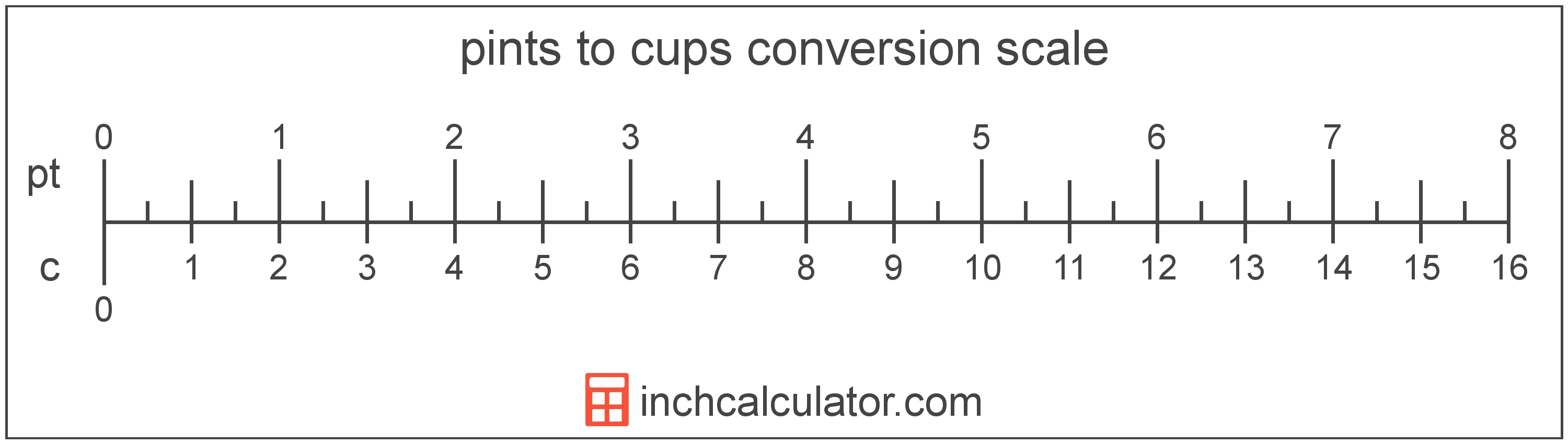Convert Cups to Pints

Enter the volume in cups below to get the value converted to pints.

Results in Pints:1 c = 0.5 pt
Do you want to convert pints to cups?

How to Convert Cups to PintsTo convert a cup measurement to a pint measurement, multiply the volume by the conversion ratio. One cup is equal to 0.5 pints, so use this simple formula to convert:

pints = cups × 0.5

The volume in pints is equal to the cups multiplied by 0.5.

For example, here's how to convert 5 cups to pints using the formula above.
5 c = (5 × 0.5) = 2.5 pt

Cups and pints are both units used to measure volume. Keep reading to learn more about each unit of measure.

Cups

The cup is a unit of volume equal to 16 tablespoons or 8 fluid ounces. The cup should not be confused with the metric cup or the teacup, which are different units of volume. One cup is equal to just under 236.6 milliliters, but in nutrition labelling, one cup is equal to 240 milliliters.

The cup is a US customary unit of volume. Cups can be abbreviated as c, and are also sometimes abbreviated as C. For example, 1 cup can be written as 1 c or 1 C.

Pints

The US liquid pint is a unit of fluid volume equal to one-eighth of a gallon, one-half of a quart, or two cups. The liquid pint should not be confused with the dry pint (US) or the imperial pint, which are different units.

The pint is a US customary unit of volume. Pints can be abbreviated as pt, for example 1 pint can be written as 1 pt.

Cup Measurements and Equivalent Pint Conversions

Common cup values converted to the equivalent pint value
Cups Pints
1 c 0.5 pt
2 c 1 pt
3 c 1.5 pt
4 c 2 pt
5 c 2.5 pt
6 c 3 pt
7 c 3.5 pt
8 c 4 pt
9 c 4.5 pt
10 c 5 pt
11 c 5.5 pt
12 c 6 pt
13 c 6.5 pt
14 c 7 pt
15 c 7.5 pt
16 c 8 pt
17 c 8.5 pt
18 c 9 pt
19 c 9.5 pt
20 c 10 pt
21 c 10.5 pt
22 c 11 pt
23 c 11.5 pt
24 c 12 pt
25 c 12.5 pt
26 c 13 pt
27 c 13.5 pt
28 c 14 pt
29 c 14.5 pt
30 c 15 pt
31 c 15.5 pt
32 c 16 pt
33 c 16.5 pt
34 c 17 pt
35 c 17.5 pt
36 c 18 pt
37 c 18.5 pt
38 c 19 pt
39 c 19.5 pt
40 c 20 pt

More Cup Volume Conversions

US Liquid
Convert to Gallons
1 c is equal to 0.0625 gallons
Convert to Quarts
1 c is equal to 0.25 quarts
Convert to Fluid Ounces
1 c is equal to 8 fluid ounces
US Cooking
Convert to Tablespoons
1 c is equal to 16 tablespoons
Convert to Teaspoons
1 c is equal to 48 teaspoons
US Customary Volume
Convert to Cubic Inches
1 c is equal to 14.4375 cubic inches
Convert to Cubic Feet
1 c is equal to 0.008355 cubic feet
Convert to Cubic Yards
1 c is equal to 0.000309 cubic yards
Metric Volume
Convert to Liters
1 c is equal to 0.236588 liters
Convert to Milliliters
1 c is equal to 236.588236 milliliters
Convert to Cubic Centimeters
1 c is equal to 236.588236 cubic centimeters
Convert to Cubic Meters
1 c is equal to 0.000237 cubic meters